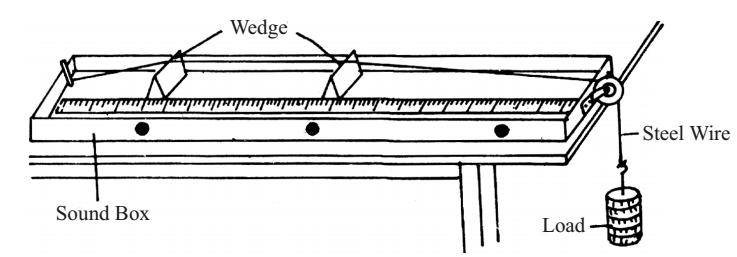# Sonometer Experiment

Experiment: To establish graphically the relation between the tension and length of the string of a sonometer resonating with a given tuning fork. Use the graph to determine the mass per unit length of the string.

A stretched string that is set into vibrations produces musical sound. When a string stretched between two points is plucked, several modes of oscillation may be present. However, the simplest mode of vibration with lowest frequency for a given length of a string is called the fundamental mode of vibration and its frequency is given by

f = 1/2L √(F/μ)

Where L is the length of the vibrating segment, F is the tension in string and n is the mass per unit length of the string. For a given frequency and a given string, f and μ are constant,

√F ∝ L (f, μ constant)

This means that the tension (F) applied to the string, varies as the square of the length (L) necessary for resonance.

In this experiment, you will establish the above relation by the use of a sonometer. A sonometer consists essentially of one or two piano wires stretched over a sounding box. The tension is applied by means of a hanger and weights suspended from each wire, by passing the wire over to a pulley and the effective length is regulated by movable bridges.Material Required

Sonometer, weight hanger and slotted weights (ten ½ kg weights), tuning fork, rubber mallet or block, meter-stick (if sonometer does not have length scale), 2 sheets of cartesian graph paper

### How To Perform Experiment

1. Place the sonometer near the end of the laboratory table so that the weight hanger attached to the wire hangs freely.

2. Set the two bridges near the ends of the wire so as to utilize most of the length. Add weights to the -weight hanger. (Caution: Keep your feet clear of the suspended weights in case the wire breaks or comes loose and the weights falls).

3. Fold a small paper strip in V-shape and place it as a rider in the middle of the string.

4. Sound the tuning fork by striking it with a rubber mallet or on a rubber block. Never strike the tuning fork against a hard object (e.g. a table).

This may damage the fork and change its characteristic frequency. Place the base of the vibrating fork on the top of the sonometer and observe the vibrations of the paper rider. Then, vary the vibrating length of the string by sliding its bridge until the string resonates with the vibrating tuning fork. This you ensure by noting that a loud sound is heard and the paper rider flutters vigorously and falls. Measure the vibrating length of the string, that is the length between the two bridges and record this length. Remember that the string vibrates in one segment in fundamental mode of vibration.

5. Change the tension in the wire by adding ½ kg weight to the hanger and repeat procedure 4.

6. Repeat the experiment for 6 or more values of tension.